Home > A2C > Chapter 8 > Lesson 8.1.2 > Problem8-31

8-31.
1. Solve each of the following equations and check your solutions. Homework Help ✎

1.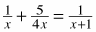Challenge: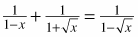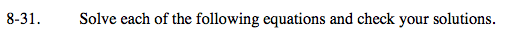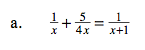Multiply by the common denominator 4x(x + 1) to remove the fractions.

x = −1.8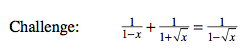$x =\frac{1}{4}$